Introduction to Analysis : Power Series

Example Questions

Example Question #1 : Power Series

Determine whether the following statement is true or false:

Some power series such ashave a possible radius of convergence that can be found by computing the roots of the coefficients of.

True

False

False

Explanation:

This statement is false because every power series has a radius of convergence that is found by computing the roots the series coefficients.

The proof of one case is as follows:

When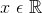is fixed,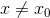, and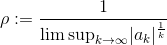with the assumption that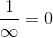and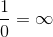the Root Test is applied to the series.

When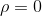by the assumption and the notation that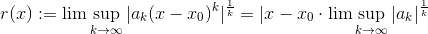implies that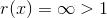therefore by the Root Test,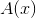does not converge for anyand thus the radius of convergence ofis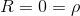.